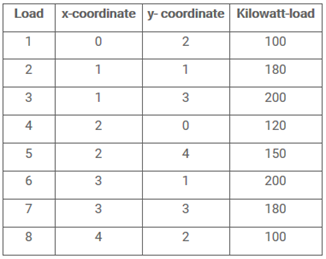# Solution: Electrical loads are arranged on horizontal x, y axes as follows

(Last Updated On: January 15, 2020)#### Problem Statement: EE Board March 1998

Electrical loads are arranged on horizontal x, y axes as follows.What is the value of x and y?

• A. x = 2.000, y = 2.049
• B. x = 2.163, y = 2.195
• C. x = 1.854, y = 2.211
• D. x = 2.146, y = 1.902